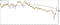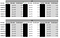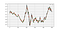# Searching for a profitable moving average crossover trading strategy in Python.

Moving averages were among the first indicators of trend-following and to this day, they remain as one of the pillars of trading. They’re not just used by technical analysts but also by traders unfamiliar with technical analysis.

Moving averages are a quick way of deciphering the trend and looking for dynamic supports and resistances. And even though, the litterature differs on the validity of the concept of supports and resistances, we will stick to the fact that they do exist and provide buying and selling levels.700-Day simple moving average acting as a dynamic support and resistance level on the AUDUSD.

We will back-test the famous crossover strategy relying on moving averages. The idea here is to try out different lookback and holding periods. For example, the most famous two sets are the 60-period and the 200-period due to their simplicity and their intuition (60 representing approximately 3 business months). As usual, we will back-test the 6 major FX pairs on different time frames this time and with different moving average settings. Our aim from this study is to see whether there is a combo that works well on different FX pairs, that is, if any combo works.

We will use the below function on an OHLC data and start looping around popular moving average combos (like the 60/200).

`def moving_average(Data, short, long):  # Short MA for i in range(len(Data)):   try:     Data[i, 4] = (Data[i — short + 1:i + 1, 3].mean())    except IndexError:     pass  # Long MA for i in range(len(Data)):   try:     Data[i, 5] = (Data[i — long + 1:i + 1, 3].mean())    except IndexError:     pass   Data = Data[long:,]  # Crossover signals for i in range(len(Data)):try:     if Data[i, 4] > Data[i, 5] and Data[i — 1, 4] < Data[i — 1, 5]:       Data[i + 1, 6] = 1 # Buy on next open     elif Data[i, 4] < Data[i, 5] and Data[i — 1, 4] > Data[i — 1, 5]:       Data[i + 1, 7] = -1 # Short on next open     else:       continueexcept IndexError:    pass`

In my recent book “The book of back-tests: Trading objectively”, I have mostly used a fixed holding period for the strategies presented. Now, instead of using a fixed holding period, we will simply be closing the position as soon as we have a contrarian signal. This means we will close the position after getting a contrarian signal. This is also known as a variable holding period.Table: Performance table showing that generally, variable holding periods do not work quite well on the tested pairs.Figure: Signal chart example on the 3H time frame EURUSD

Interesting configurations that arised while conducting back-tests:

• EURUSD 10/20 moving averages on a weekly level had a 44.00% hit ratio with a 2.20 profit factor. This signifies that over the past, this strategy did provide above average returns. In fact, a trader using 0.1 lots and starting on the first trading day of January 2000 would have gained \$10,327 on a \$1,000. This amounts to \$100,327 with a standard lot.
• USDCAD 20/60 moving averages on a daily level had a 44.44% hit ratio with a 1.41 profit factor. This signifies that over the past, this strategy did provide above average returns. In fact, a trader using 0.1 lots and starting on the first trading day of January 2000 would have gained \$5,446 on a \$1,000. This amounts to \$5,446 with a standard lot.

Now, the above back-tests were indeed disappointing and especially coming from a known indicator such as the moving average.

What if we try another holding period method? after all, the strategy’s signals are what they are, but we can still control for risk management. We do not want to tweak the lookback periods too much (e.g. short moving average at 19 and long moving average at 73) so as to avoid overfitting. Something simple that works on average for most currency pairs has potential and that is exactly what we should look for. So, we will re-do the above back-test only with standard fixed holding periods instead of variable holding periods. We will use

The notable result here lies only with the NZDUSD which has delivered acceptable results in the past. The EURUSD is in blue to highlight the difference between the results here and the results in the Book of Back-tests; the holding period used in the book was 7 while here was 100.

Now, if we take the NZDUSD and try to spuriously optimize it (which I do not recommend as it encourages overfitting) we find that the optimal parameters are:

• Holding period: 52
• Short moving average: 13
• Long moving average: 103

Giving us the below optimized equity curve (even after deducting transaction costs):

# Conclusion

Moving averages remain one of the easiest and most versatile indicators in analysis. They have a lot of potential and can be optimized and also combined with other technical indicators to form a more robust strategy. Risk management can be added to the strategy while accounting for volatility as well. It requires intensive back-tests and sound statistical significance tests to find the optimal and suitable strategy for each individual. The main points derived here were:

• Trying different types of holding periods can help detecting hidden strategies. Holding periods can be fixed, variable based on contrarian signals, variable based on a form of volatility measure (such as the ATR), or any other customized variation. I always prefer the simpler measures.
• We can use a wide array of moving averages to form strategies that use simple crossovers or even more complex forms of crossovers.
• It is not recommended to tweak the parameters too much to optimize the past as the future is unlikely to be exactly the same. Instead, we have to focus on finding strategies that work well on most assets and are not sensitive to a small change in inputs.
• Searching for profitable strategies should begin first with the intuition before actually conducting the back-test. In here, we have made the theory that a crossover might indicate a change in trend, but what are the good parameters that allow us to form a good strategy? That’s a different story that requires more work.
• It seems that longer-term moving averages with longer-term fixed holding periods deliver better results than the rest.

Written by